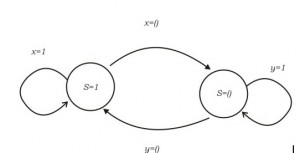Related Articles

# GATE | GATE IT 2006 | Question 37

• Last Updated : 28 Jun, 2021

For a state machine with the following state diagram the expression for the next state S+ in terms of the current state S and the input variables x and y is(A) S+ = S’ . y’ + S . x
(B) S+ =S. x . y’ + S’ . y . x’
(C) S+ =x . y’
(D) S+ =S’ . y + S . x’col

Explanation:

For the next state to be S=1 there are two possible cases:

• S=1, x=1
• S=0, y=0

For the next state to be S=0 there are two possible cases:

• S=1, x=0
• S=0, y=1

The above conditions can be tabulated as (where X means that variable has no effect on state transition)

 x y S S+ 1 X 1 1 X 0 0 1 0 X 1 0 X 1 0 0

It is clearly visible that S+ depends on state S and either of the variable x or y. This directly eliminates option (B) and option (C).
Now we can check for the other two options. When S=1 and x=1 option (D) gives S+ as 0 which should otherwise be one hence (D) can also be eliminated.
Option (A) satisfies all, hence the answer.

This solution is contributed by Yashika Arora.

Quiz of this Question

Attention reader! Don’t stop learning now.  Practice GATE exam well before the actual exam with the subject-wise and overall quizzes available in GATE Test Series Course.

Learn all GATE CS concepts with Free Live Classes on our youtube channel.

My Personal Notes arrow_drop_up